##Desargues involution theorem in the complex projective space

The theorem of Desargues for families of conics passing through four points (see DesarguesInvolution.html ) is valid also for conics in the complex projective space PC2.
The formal definition and the proof of the theorem are the same as in the real case.
A particular case arises for the family of real conics generated by two (real) conics c1, c2 which have no real intersection point at all. For any line (e), though, the intersections of the member conics with the line define point X1, X2 in involution.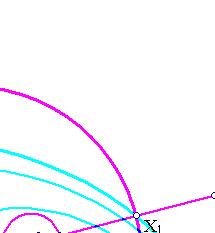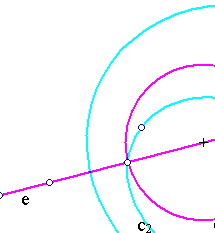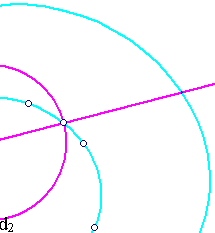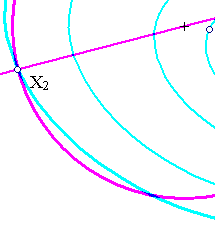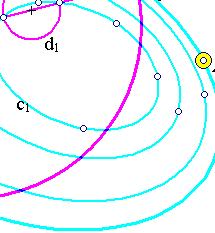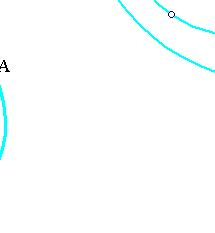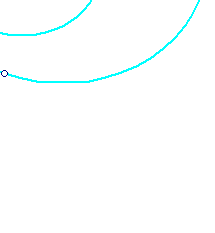The proof results from the theorem in the complex projective plane, by considering the conics c1 and c2 as conics of that plane. The circles (d) with diameters X1X2 on line (e) build a circle bundle. This is again a particular case of the above theorem.

### See Also

CrossRatio0.html
DesarguesInvolution.html
DesarguesInvolution2.html
HomographicRelation.html
Involution.html
InvolutionBasic.html
InvolutionBasic2.html

 Produced with EucliDraw© http://users.math.uoc.gr/~pamfilos/